# Maharashtra Board Class 9 Maths Solutions Chapter 7 Statistics Practice Set 7.4

## Maharashtra State Board Class 9 Maths Solutions Chapter 7 Statistics Practice Set 7.4

Question 1.
Complete the following cumulative frequency table: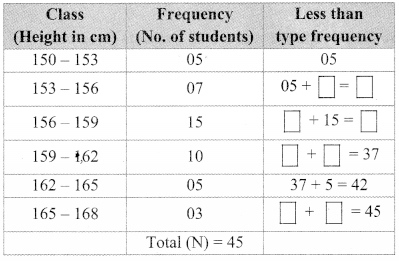Solution:Question 2.
Complete the following Cumulative Frequency Table:Solution: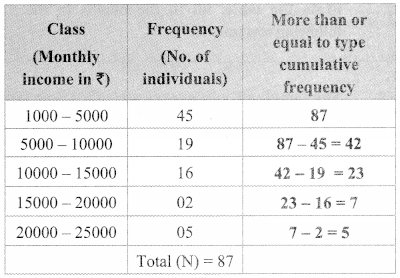Question 3.
The data is given for 62 students in a certain class regarding their mathematics marks out of 100. Take the classes 0 – 10, 10 – 20,… and prepare frequency distribution
table and cumulative frequency table more than or equal to type.
55. 60, 81, 90, 45, 65, 45, 52, 30, 85, 20, 10,
75, 95, 09, 20, 25, 39, 45, 50, 78, 70, 46, 64,
42. 58. 31, 82, 27, 11, 78, 97, 07, 22, 27, 36,
35, 40, 75, 80, 47, 69, 48, 59, 32, 83, 23, 17,
77, 45, 05, 23, 37, 38, 35, 25, 46, 57, 68, 45.
47,49
From the prcparcd table, answer the following questions :
i. How many students obtained marks 40 or above 40?
ii. How many students obtained marks 90 or above 90?
iii. How many students obtained marks 60 or above 60?
iv. What is the cumulative frequency of equal to or more than type of the class 0 – 10?
Solution: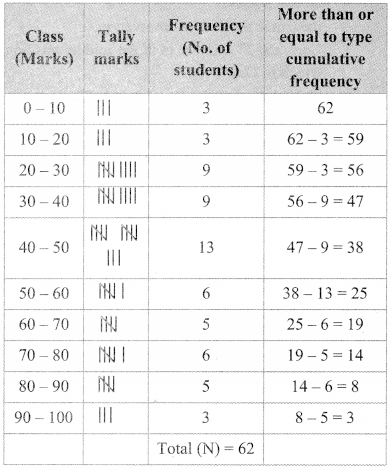i. 38 students obtained marks 40 or above 40.
ii. 3 students obtained marks 90 or above 90.
iii. 19 students obtained marks 60 or above 60.
iv. Cumulative frequency of equal to or more than type of the class 0 – 10 is 62.

Question 4.
Using the data In example (3) above, prepare less than type cumulative frequency table and answer the following questions.
i. How many students obtained less than 40 marks?
ii. How many students obtained less than 10 marks?
iii. How many students obtained less than 60 marks?
iv. Find the cumulative frequency of the class 50 – 60.
Solution: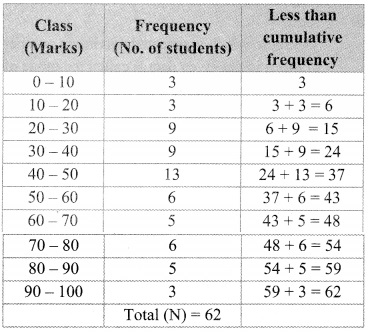i. 24 students obtained less than 40 marks.
ii. 3 students obtained less than 10 marks.
iii. 43 students obtained less than 60 marks.
iv. Cumulative frequency of the class 50 – 60 is 43.

Maharashtra Board Class 9 Maths Chapter 7 Statistics Practice Set 7.4 Intext Questions and Activities

Question 1.
The following information is regarding marks in mathematics, obtained out of 40, scored by 50 students of 9th std. ¡n the first unit test. (Textbook pg. no. 120)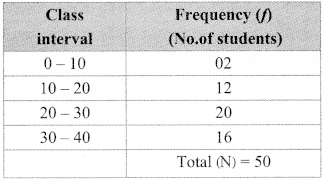From the table, fill in the blanks in the following statements.
i. For class interval 10 – 20 the lower class limit is _____ and upper class limit is _____
ii. How many students obtained marks less than 10? 2
iii. How many students obtained marks less than 20? 2 + ____ = 14
iv. How many students obtained marks less than 30? ______ + _____ = 34
v. How many students obtained marks less than 40? ______ + ______ =50
Solution:
i. 10, 20
iii. 12
iv. 14 + 20
v. 34 + 16

Question 2.
A sports club has organised a table-tennis tournaments. The following table gives the distribution of players ages. Find the cumulative frequencies equal to or more than the lower class limit and complete the table (Textbook pg. no. 121)
Solution:
Equal to lower limit or more than lower limit type of cumulative table.